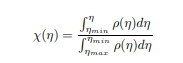# Cumulative variable

I have an eta distribution that I want to convert to a distribution of a new cumulative variable Chi(eta), so that the new distribution becomes flat.
I can’t figure out the block of code that can be used for cumulative functions.
Thank you.ROOT Version: v6
Platform: ubuntu 20

Do you have some code already ? if yes can you post it ?

1 Like

I can only scale the histogram like this:

``````    TH1F *ratio11 = (TH1F*)h5->Clone()

Float_t norm11 = 1;
Float_t scaleeta11 = norm11/(ratio11->Integral());
ratio11->Scale(scaleeta11);
``````

I have no idea on how to use this function and also the integration limits.

Hi,

The function `TH1::GetIntegral()` is probably what you are looking for. See ROOT: TH1 Class Reference.

Lorenzo

1 Like

Thank you for the reply. How should I use GetIntegral() for the above formula?

`TH1::GetIntegral()` implement exactly the above formula.
if `fEtaBin1` is your original histogram, you cumulative histogram is obtained as following:

``````auto ig = fEtaBin1->GetIntegral()
auto cumHist = new TH1D("cumHist","cumulative distribution",fEtaBin1->GetNbinsX(), fEtaBin1->GetXaxis()->GetXmin(),fEtaBin1->GetXaxis()->GetXmax());
for (int i = 1; i <= cumHist->GetNbinsX(); i++)
cumHist->SetBinContent(i, ig[i-1] );

cumHist->Draw();
``````
1 Like

Hi,

I don’t think so. The cumulative distribution should be between 0 and 1. I see instead there a uniform distribution at ~ 35E6. Please post the full code, so we can understand what went wrong

Lorenzo

1 Like